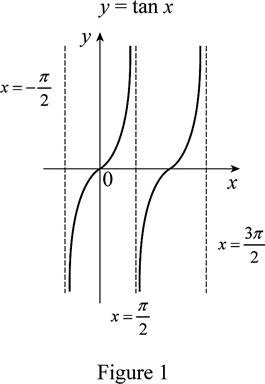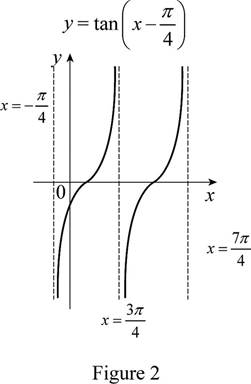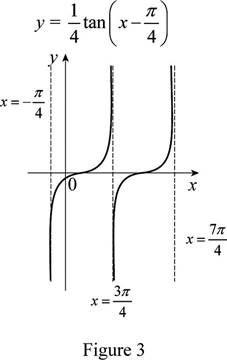# The function y = tan x by hand without plotting any points and then graph of the function y = 1 4 tan ( x − 1 4 ) .### Single Variable Calculus: Concepts...

4th Edition
James Stewart
Publisher: Cengage Learning
ISBN: 9781337687805### Single Variable Calculus: Concepts...

4th Edition
James Stewart
Publisher: Cengage Learning
ISBN: 9781337687805

#### Solutions

Chapter 1.3, Problem 22E
To determine

## To sketch: The function y=tanx by hand without plotting any points and then graph of the function y=14tan(x−14) .

Expert Solution

### Explanation of Solution

The standard graph of the function y=tanx is roughly drawn as shown below in Figure 1.Then, draw the graph of y=tan(x14) , shift the graph y=tanx by π4 units to the right. Thus, the graph of y=tan(x14) is shown below in Figure 2.From Figure 2, it is observed that Figure 1 is shifted π4 units to the right. Then, draw the graph of y=14tan(x14) , shrunk the graph y=tan(x14) vertically by a factor of 4. Thus, the graph of y=14tan(x14) is shown below in Figure 3.Observe that Figure 3 is obtained from Figure 1 in such a way that it is shifted π4 units to the right and then compressed vertically by a factor of 4.

### Have a homework question?

Subscribe to bartleby learn! Ask subject matter experts 30 homework questions each month. Plus, you’ll have access to millions of step-by-step textbook answers!